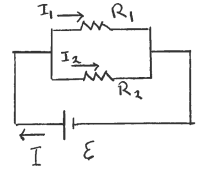# Problem: The circuit shown in the sketch consist of two resistors and a battery with emf ε = 24.0 V and negligible internal resistance, R1 = 2.00 Ω and R2 = 4.00 Ω. What is the current through each resistor and what current I flows through the battery? I1 =  I2 =  I3 =

81% (10 ratings)
###### Problem Details

The circuit shown in the sketch consist of two resistors and a battery with emf ε = 24.0 V and negligible internal resistance, R1 = 2.00 Ω and R2 = 4.00 Ω. What is the current through each resistor and what current I flows through the battery?

I1 =

I2 =

I3 =